# Tangent and Normal

## Application of derivatives of Class 12

Let y = f(x) be the equation of a curve and let P(x0, y0) be a point on the curve. Let PT be the tangent, PN the normal and PM be perpendicular to the axis of x.

The slope of the tangent to the curve y = f(x) at P is given by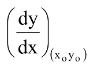and therefore the equation to the tangent to the curve y = f(x) at (x0, y0) is y −y0 =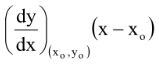and the equation to the normal will be y −y0 +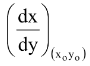(x −xo) = 0 Length of the tangent is PT = y0 cosecθ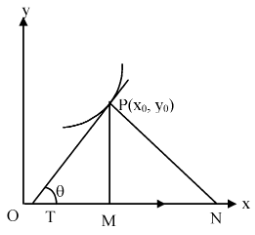= y0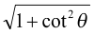= y0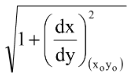and length of the normal is

y0 secθ = y0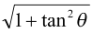= y0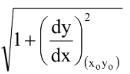Likewise lengths of the subtangent and subnormal can also be calculated.